# Model Development and Sufficiency Results

 < Day Day Up >

### Background

Assume that there exists a convex differentiable loss function l(x) on a compact convex decision set D Rn. We assume that l(x) has a unique global minimum of zero. Each of a sample of i = 1,..., N experts provides an estimate xi for x*, the ideal point of l(x), in such a way that the values li=l(xi) are distributed according to a density, g(x), here called the expert performance density, on the range of l(x). Let SD(l0)={x: l(x)=l0} D. Assume that the distribution of x given l0, that is, the density of x on SD (l0), is uniform. We call this the contour density. This last assumption asserts that estimates of the same loss value are distributed with equal probability above and below the minimizer of l(x), called the ideal point.

Next let f(x) be the corresponding density of the xi when such a density exists. We will refer to f(x) as the expert estimate density. Note that the sample observations xi relate to statistical properties of f(x). Now define AD(l) to be the volume measure in Rn of the set TD (l) = {x: l(x) l} D. That is, AD(l) is the Lebesgue measure in Rn of the set TD(l). Finally denote the range of l(x) on D by the interval [a, b] and assume that AD(l) is differentiable. For the forecasting combination application we consider, D will be all of the real line, and [a, b] becomes [0, ]. Then the first result is

Theorem 1: Consider the process given by first selecting a value of 1 in [a, b] according to a specified density g(x), andthen selecting x uniformly on the set SD(l). If the density for x exists, it is given by

 (1)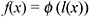where

 (2)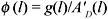Proof: This proof is a modification of a similar result in Troutt (1993). By the uniform contour density assumption, f(·) must be constant on the level curves of l(x). It follows that f(x) = φ (l(x)) for some function φ . Let G(l) be the cumulative distribution function for l. For l [a, b] and ε sufficiently small

 (3)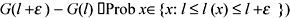Division by ε and passage to the limit yields

 (4)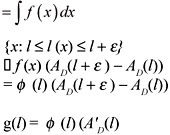and hence (2).

Evidently, a sufficient condition that the mode of f(x) coincides with the minimizer of l(x) is that φ (x) be monotone decreasing and assuming differentiability, then

 (5)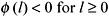The positivity of φ (l(x)) = f(x)) requires that A'(l)0. Next taking the logarithmic derivative of (4) yields

 (6)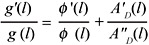and therefore

 (7)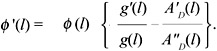Since φ (l) =f(x) 0, we therefore have proven

Theorem 3: The mode of the f(x) density (expert estimates) identifies the minimum point of l(x) if

 (8)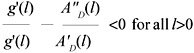Condition (8) shows the required relationship between the loss function, l(x), and the expertise density, g(l) in order that the mode of f(x) identifies the most accurate estimate. The next two examples illustrate its use.

Example 1: Suppose kl and k2 > 0 and let

 (9)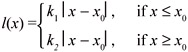Let g(l) be an arbitrary differentiable monotone decreasing density on l 0. (For example, g(l) = μe-μ1, μ > 0.) Here A(l) = (1/k1 + l/k2) with A "(l) = 0. Hence (9) holds since g'(l)<0.

Example 2: Let l(x) = k(x - x0)2 and suppose g(l) = μe-μ1, μ > 0. Hence A(l) = 2(l/k)1/2, with A'(l) = (l/k)1/2(1/k) = k1/2l-1/2 and A"(l) = -1/2k-1/2l-3/2. Thus

 (10)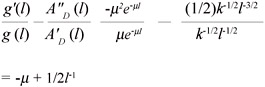for any μ > 0 there exist values of l such that (10) will be positive. Therefore the ideal point cannot be identified by the mode of the expert estimates. In fact, the density, f(x), for this case can be derived using (2) as f(x) = µ|x| exp{-μx2} which is easily seen to be bimodal.

To summarize, the mode of expert estimates will tend to identify the most accurate estimate under a wide variety of loss functions, l(x) provided that

1. Estimates of equal l(x) value, l, are uniformly distributed on the set {x: l(x)= l}. In the case of convex l(x) functions, there are two such values, each of equal likelihood.

2. Condition (8) holds.

These results suggest that, in general, the mode of individual forecasts should be expected to be an effective and simple maximum likelihood method for forecast combination. It has the potential advantage over the simple average forecast in that the l(x) function may be skewed from trial to trial, even though the performance density may be essentially constant. In "Individual and Combination Forecasts" and "Bayesian Approach," a particular mode estimator, the classical Pearson mode estimate, is obtained in a Markov chain Monte Carlo framework. The results are then applied and compared to five individual and other combination methods.

 < Day Day Up >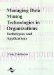Managing Data Mining Technologies in Organizations: Techniques and Applications
ISBN: 1591400570
EAN: 2147483647
Year: 2003
Pages: 174